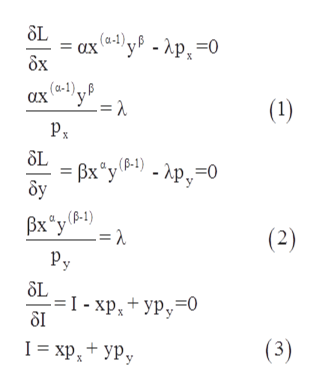# Derive demand functions for x and y for the general class of Cobb-Douglas preferences represented by the utility function u(x, y) = x α y β . As functions of px, py, I, α, and β, calculate price elasticities, cross-price elasticities, income elasticities, and expenditure shares for x and y.

Question
15 views

Derive demand functions for x and y for the general class of Cobb-Douglas preferences represented by the utility function u(x, y) = x α y β . As functions of px, py, I, α, and β, calculate price elasticities, cross-price elasticities, income elasticities, and expenditure shares for x and y.

check_circle

Step 1

The utility function of Cobb-Douglas preferences is given as,

Step 2

Derivation the demand functions for x and y

Maximize the given utility function subject to budget constraint using Lagrange method.

Step 3

First order conditions ...help_outlineImage TranscriptioncloseSL = ax (-) y® - ^p, =0 бх ах в-)ур = 1 (1) Px SL — Вх"у в) - Хр, 30 бу - Ap,=0 Вх "у (4) = 1 (2) Py SL %3D1-хр, + ур, -0 SI (3) 1- хр, + уру fullscreen

### Want to see the full answer?

See Solution

#### Want to see this answer and more?

Solutions are written by subject experts who are available 24/7. Questions are typically answered within 1 hour.*

See Solution
*Response times may vary by subject and question.
Tagged in